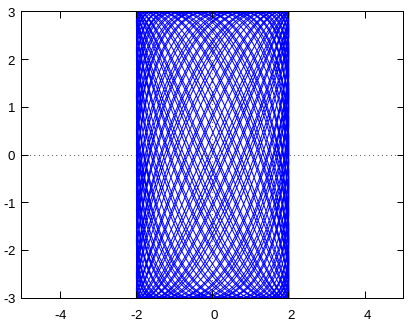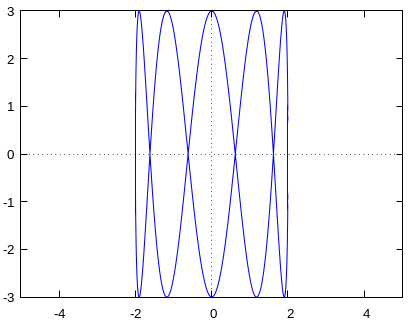# Consider the parametric curve: x(t) = 2 cos(\sqrt 2 t) y(t) = 3 sin(\sqrt 3 t) 0 \leq t < n\pi As...

## Question:

Consider the parametric curve:

{eq}\begin{matrix} x(t) = 2 \cos (\sqrt 2 t)\\ y(t) = 3 \sin(\sqrt 3 t) \end{matrix} 0 \leq t < n\pi {/eq}

As the value for {eq}n \to \infty {/eq}, what set of points in the xy-plane will be it by the curve?

Now change the coefficient of an angle:

{eq}\begin{matrix} x(t) = 2 \cos (\sqrt 2 t)\\ y(t) = 3 \sin(\sqrt{11} t) \end{matrix} 0 \leq t < n\pi {/eq}

Finally, experiment with the curve

{eq}\begin{matrix} x(t) = 2 \cos(\sqrt 2 t)\\ y(t) = 3 \sin(\sqrt a t) \end{matrix} 0 \leq t < n\pi {/eq}

Determine at least 4 values for a such that this parametric curve is vastly different from the previous examples. Hypothesize what the relationship is between 2 and a that creates this difference in results.

## Parametric Curves; Periodicity:

The trigonometric functions {eq}\sin(t) {/eq} and {eq}\cos(t) {/eq} are periodic and have the same period {eq}2\pi {/eq}. The functions {eq}\sin(at) {/eq} and {eq}\cos(bt) {/eq} have a common period if the coefficient {eq}a {/eq} is a rational multiple of {eq}b {/eq} or vice-versa in which case the parametric curve {eq}(\sin(at),\;\cos(bt)) {/eq} forms a closed loop. Otherwise there is no finite time where the curve retraces itself,

We see that the curves,

{eq}\begin{align*} & \left \{ \begin{matrix} x(t) = 2 \cos (\sqrt 2 t)\\ y(t) = 3 \sin(\sqrt{3} t) \end{matrix}\right .\\ &\text{and}\\ & \left \{ \begin{matrix} x(t) = 2 \cos (\sqrt 2 t)\\ y(t) = 3 \sin(\sqrt{11} t) \end{matrix}\right . \end{align*} {/eq}

for {eq}n {/eq} a large integer, have graphs for {eq}0<t<n\pi {/eq} similar to the one shown below (blue color).The curve traced seems to try to fill the whole rectangle {eq}[-2,2]\times[-3,3]. {/eq}

Now we consider

{eq}\begin{align*} \left \{\begin{matrix} x(t) = 2 \cos (\sqrt 2 t)\\ y(t) = 3 \sin(\sqrt{k^2\cdot 2} t) \end{matrix}\right .&\text{ for }k=2,3,4,5\cdots \end{align*} {/eq}

For these curves we obtain simple, self intersecting, loops

like the one shown below for the case {eq}k=5: {/eq}So the for values of

{eq}\boxed{a\in\{\sqrt{k^2\cdot 2}\;|\;k=2,3,4,5\}=\{2\sqrt{2},\;3\sqrt{2},\;4\sqrt{2},\;5\sqrt{2}\}} {/eq} we obtain curves that do not fill the rectangle as the ones given in the problem statement do.

This is because {eq}\sqrt{k^2\cdot 2}=\sqrt{2}\,k {/eq} is an integer multiple of {eq}\sqrt{2} {/eq} so the two coordinate functions share a common period and retrace themselves as the variation of {eq}t {/eq} is allowed over arbitrarily long periods.